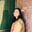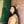Trusted answers to developer questions
Trusted Answers to Developer Questions

Related Tags

numpy
python

# What is numpy.amax() in Python?Umme Ammara

Grokking Modern System Design Interview for Engineers & Managers

Ace your System Design Interview and take your career to the next level. Learn to handle the design of applications like Netflix, Quora, Facebook, Uber, and many more in a 45-min interview. Learn the RESHADED framework for architecting web-scale applications by determining requirements, constraints, and assumptions before diving into a step-by-step design process.

Python’s numpy.amax() computes the maximum of an array or of an array along a specified axis.

## Syntax

numpy.amax() is declared as follows:

numpy.amax(a, axis=None, out=None, keepdims=<no value>, initial=<no value>, where=<no value>)


In the syntax given above, a is the non-optional parameter, and the rest are optional parameters.

## Parameters

numpy.amax() takes the following non-optional parameter:

• a [array-like] - input array.

numpy.amax() takes the following optional parameters:

• axis [None, int, tuples of int] - axis along which we want the maximum value to be computed. The default is the flattenedinput converted from multi-dimensional to a one-dimensional array array.

• out [ndarray] - represents the location into which the output is stored.

• keepdims [boolean] - True value ensures that the reduced axes are left as dimensions with size one in the output. This ensures that the output is broadcasted correctly against the input array. If a non-default value is passed, keepdims will be passed through to the amax() method of sub-classes of ndarray. In the case of the default value, this will not be done.

• initial [scalar] - minimum value of the output element.

• where [array_like of bool] - represents the elements to compare for the maximum.

## Return value

numpy.amax() returns the maximum of an array. The return type is ndarray or scalar, depending on the input.

• If an axis is specified, the output is an array of dimension a.ndimthe number of array dimensions - 1.

• If an axis is None, the output is scalar.

• If one or both of the values being compared is NaN (Not a Number), NaN is returned.

## Examples

The following example outputs the maximum value of the array arr where the axis parameter is not specified:

import numpy as nparr = np.array([1,2,5,6,0])print(np.amax(arr))

The example below outputs the maximum of the array arr1 where axis is specified as 0 and 1.

import numpy as nparr1 = np.array([[2,4,5], [7,10,1]])#Maximum values along the first axisprint(np.amax(arr1, axis = 0))#Maximum values along the second axisprint(np.amax(arr1, axis = 1))

RELATED TAGS

numpy
python

CONTRIBUTORUmme Ammara